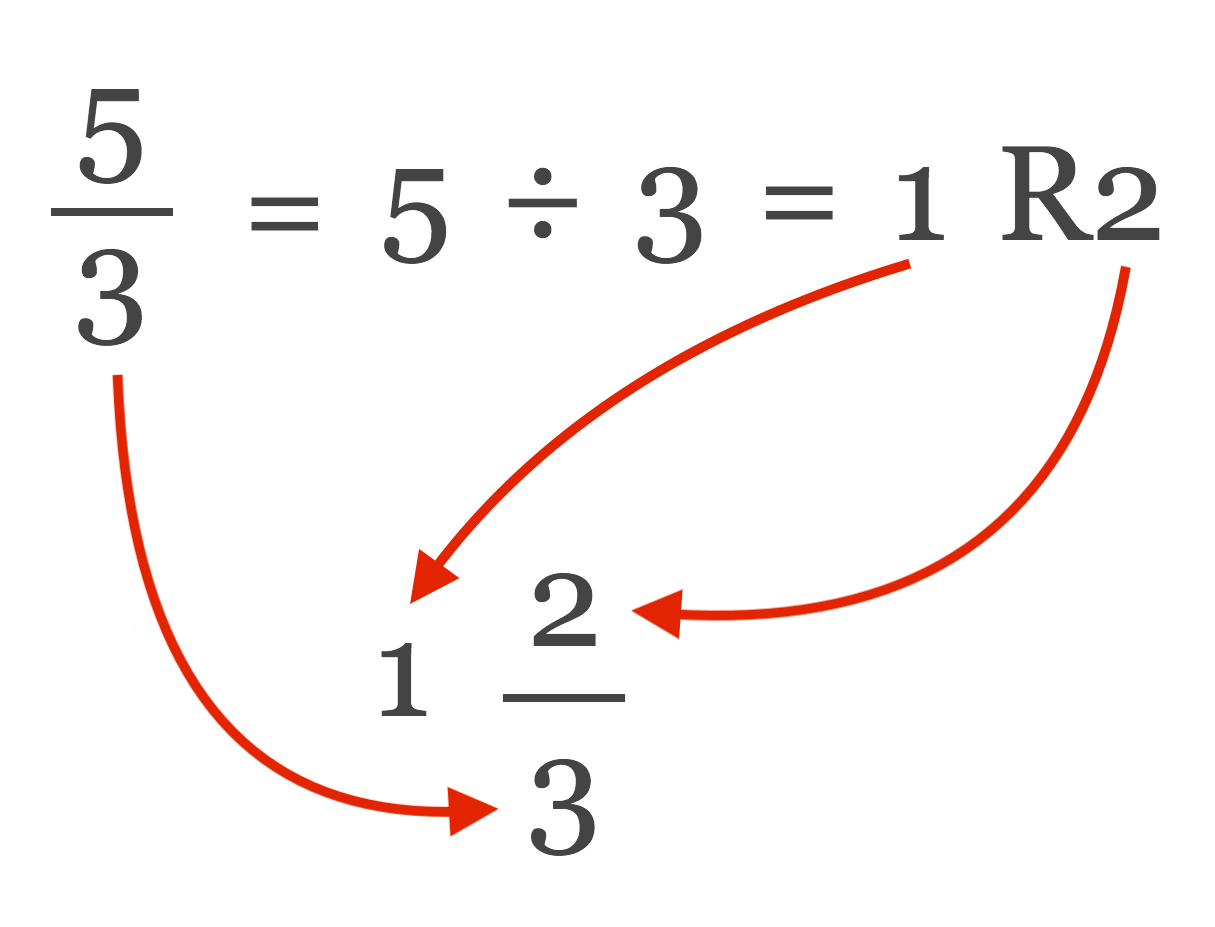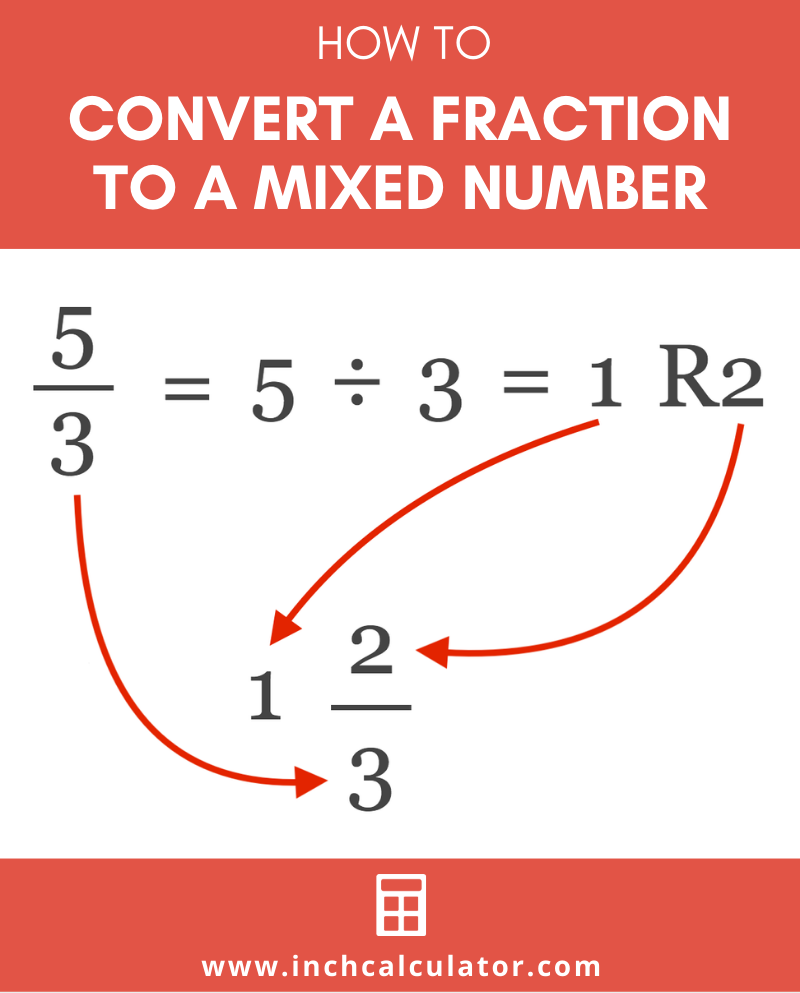# Fraction to Mixed Number Calculator

Enter the improper fraction in the fields below to convert it to a mixed number. The calculator shows all the work so you can follow along and learn the steps.

Fraction:

## Mixed Number:

12 / 3

### Steps to Convert to a Mixed Number

Convert the fraction to a mixed number by using long division to find the quotient and remainder
5÷3=1R2
The quotient will be the whole number in the fraction, and the remainder will be the numerator in the mixed fraction
5 / 3=12 / 3
Learn how we calculated this below

You might also like our calculator to convert a mixed number to an improper fraction.

## How to Convert an Improper Fraction to a Mixed Number

An improper fraction is a fraction with no whole number and has a larger numerator than the denominator. We can simplify these fractions to mixed numbers in a few simple steps.### Step One: Use Long Division

The first step in the conversion is to use long division to find the quotient and the remainder. These will be used in the next step.

When doing long division with a fraction, the numerator will be the dividend, and the denominator will be the divisor.

For example, let’s solve the quotient and remainder of 7 / 3.

7 / 3 = 7 ÷ 3
7 ÷ 3 = 2 R1

Thus, the quotient is 2, and the remainder is 1.

### Step Two: Rewrite the Quotient and Remainder to the Mixed Number

The second step is to rewrite the fraction as a mixed number using the quotient and remainder from the previous step, along with the original denominator.

To convert, set the quotient as the whole number, the remainder as the numerator, and the original denominator as the denominator.

For example, let’s use the quotient and remainder from the previous step to rewrite 7 / 3 as a mixed number.